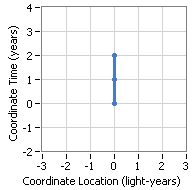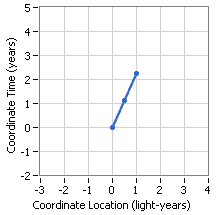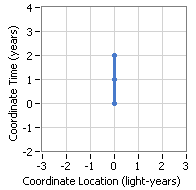# Confused with Special Relativity

tolove
If light takes 1 year to reach a destination, and it takes a ship 2 years to reach the same destination, how fast is the ship going?

Is a question like this possible to answer without more information? I'm looking at the Lorentz transformations, and am finding all sorts of ways to be confused. The equations are undefined when v = c, and distances change in the moving frame depending on the values of v.

What is the distance for between points for light? Does this question have meaning?

Gold Member
If light takes 1 year to reach a destination, and it takes a ship 2 years to reach the same destination, how fast is the ship going?
One-half the speed of light. Why isn't this obvious to you?
Is a question like this possible to answer without more information? I'm looking at the Lorentz transformations, and am finding all sorts of ways to be confused. The equations are undefined when v = c, and distances change in the moving frame depending on the values of v.

What is the distance for between points for light? Does this question have meaning?
Like you said, distances change in different frames. You just have to do the calculations to see what they are. But you never transform to the speed of light.

Mentor
If light takes 1 year to reach a destination, and it takes a ship 2 years to reach the same destination, how fast is the ship going?
That one really is as easy as it looks: one-half the speed of light. Relativity doesn't contract the idea that speed=distance traveled divided by travel time, it just tells us that we have to be careful about mixing up different observers' time and distant measurements. In this problem we don't have to worry about that because we're only using your measurements of time and distance.

Is a question like this possible to answer without more information? I'm looking at the Lorentz transformations, and am finding all sorts of ways to be confused. The equations are undefined when v = c, and distances change in the moving frame depending on the values of v.
The Lorentz transformations tell us how to calculate, from your description of the situation, how some other observer moving relative to you will describe the same situation. The ##v## in the Lorentz transformations has nothing to do with the speed of the spaceship (unless you decide to put your "some other observer" in the spaceship, in which case the speed of the spaceship relative to you is also the speed of this other observer relative to you).

What is the distance for between points for light? Does this question have meaning?
That question has no meaning, because no observer can travel at the speed of light. However, if you're watching a flash of light zip by, you have a perfectly good notion of the distance between the point where the light was emitted and where it was received, and the time it took for the light to cover that distance, and when you divide the latter into the former you will get c, the speed of light, every time.

tolove
One-half the speed of light. Why isn't this obvious to you?

Like you said, distances change in different frames. You just have to do the calculations to see what they are. But you never transform to the speed of light.

Not much is obvious to me! I learned from your post, but let me reword the question and ask again:

A destination is 1 light year away as measured from a resting frame. A ship experiences two years of time to get to the same destination. How fast is the ship moving?

I keep getting two unknowns in all my equations, so I think I'm having a misunderstanding somewhere.

Thank you for the help!

Mentor
A destination is 1 light year away as measured from a resting frame. A ship experiences two years of time to get to the same destination. How fast is the ship moving?

That is a different question than the question you asked in post #1.

In post #1 you seemed to be saying that it took the ship two years to reach its destination as measured in the same frame in which it took the light signal one year to make the journey... and in that case, because the distance and time are both being measured in the same frame, it really is as easy as ##speed=distance/time## - the distance in that frame is one light year, the travel time is two light years, the speed comes out to .5c when you do the obvious division.
(Note that this is so easy because you aren't mixing times and distances from different frames).

But now you're asking a different question... now the travel time is two years as viewed by an observer on the spaceship, but the distance is one light-year as viewed by an observer moving relative to the spaceship. (Remember, that from the spaceship's point of view, it is the rest frame, floating in empty space while the destination is rushing towards it, and the observer in what you've been calling the "resting frame", the one who measured the one-lightyear distance to the destination, is receding behind the spaceship).

Basically, I think the question you are asking is, what speed would I have to launch a spaceship at if I wanted its crew to experience/age by two years on a journey to a destination one light-year?

Last edited:
Gold Member
Not much is obvious to me! I learned from your post, but let me reword the question and ask again:

A destination is 1 light year away as measured from a resting frame. A ship experiences two years of time to get to the same destination. How fast is the ship moving?

I keep getting two unknowns in all my equations, so I think I'm having a misunderstanding somewhere.

Thank you for the help!
You can use the Lorentz Transformation to solve this problem. We start with the rest frame of the ship taking 2 years to get to its destination:The event we care about is the arrival with coordinates of x=0 and t=2.

Now we want to transform at some unknown speed such that the event has an x' coordinate of 1. We don't know or care about t'. The new frame will look something like this:We use this LT equation:

x' = (x-tβ)/√(1-β2)

Substituting all our known values we get:

1 = (0-2β)/√(1-β2)
1 = -2β/√(1-β2)

Squaring both sides:

1 = 4β2/(1-β2)

Rearranging:

1-β2 = 4β2

Combining:

1 = 4β2 + β2
1 = 5β2
1/5 = β2
β = √0.2 = 0.447

#### Attachments

Mentor
You can use the Lorentz Transformation to solve this problem. We start with the rest frame of the ship taking 2 years to get to its destination:

There's an easier way though:
We have two events, the takeoff and the landing.

In the ship frame, the ##(x,t)## coordinates of the takeoff are ##(0,0)## and the coordinates of the landing are ##(0,2)##.

In the rest frame, the ##(x,t)## coordinates of the takeoff are ##(0,0)## and the coordinates of the landing are ##(1,q)## where ##q## is the travel time in the rest frame.

The spacetime interval between the two events is given by ##s^2=\Delta{t}^2-\Delta{x}^2##, and must be the same in both frames.

So we have ##2^2-0^2 = q^2-1^2## or ##q^2=5##; in the rest frame ship traveled one light year in ##\sqrt{5}## years so its speed is ##1/\sqrt{5} = \sqrt{.2}##

tolove
You can use the Lorentz Transformation to solve this problem. We start with the rest frame of the ship taking 2 years to get to its destination:The event we care about is the arrival with coordinates of x=0 and t=2.

Now we want to transform at some unknown speed such that the event has an x' coordinate of 1. We don't know or care about t'. The new frame will look something like this:We use this LT equation:

x' = (x-tβ)/√(1-β2)

Substituting all our known values we get:

1 = (0-2β)/√(1-β2)
1 = -2β/√(1-β2)

Squaring both sides:

1 = 4β2/(1-β2)

Rearranging:

1-β2 = 4β2

Combining:

1 = 4β2 + β2
1 = 5β2
1/5 = β2
β = √0.2 = 0.447

Heya, where are you getting that equation from?

x' = (x-tβ)/√(1-β^2)

The one in my textbooks is: x' = (x-tv)/√(1-β^2)

Also, thank you, Nugatory, for that method. It wasn't covered in my text/class either.